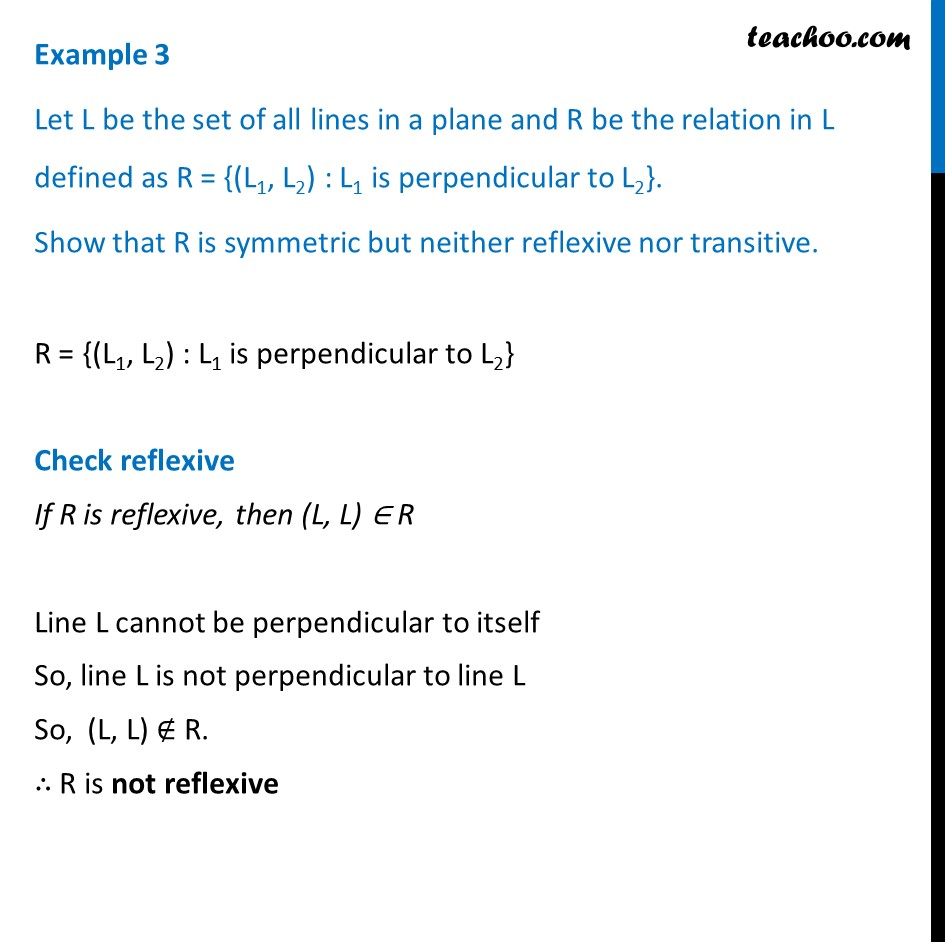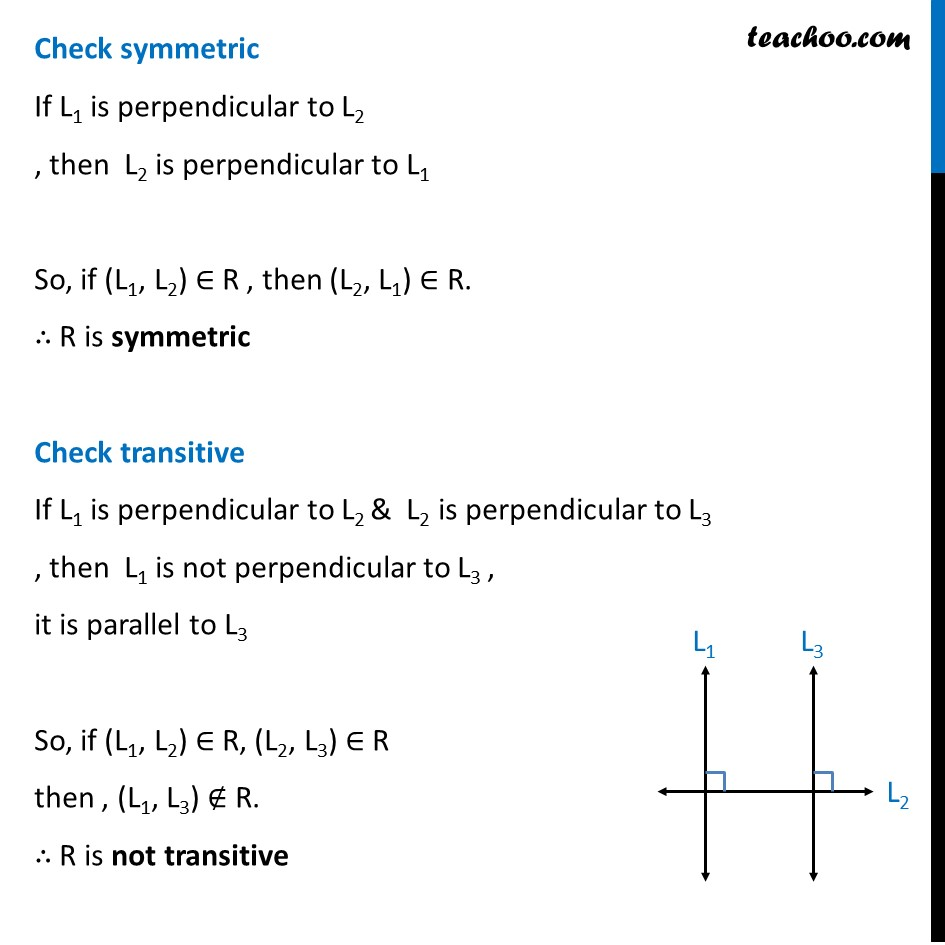Examples

Chapter 1 Class 12 Relation and Functions
Serial order wiseLearn in your speed, with individual attention - Teachoo Maths 1-on-1 Class

### Transcript

Example 3 Let L be the set of all lines in a plane and R be the relation in L defined as R = {(L1, L2) : L1 is perpendicular to L2}. Show that R is symmetric but neither reflexive nor transitive. R = {(L1, L2) : L1 is perpendicular to L2} Check reflexive If R is reflexive, then (L, L) ∈ R Line L cannot be perpendicular to itself So, line L is not perpendicular to line L So, (L, L) ∉ R. ∴ R is not reflexive Check symmetric If L1 is perpendicular to L2 , then L2 is perpendicular to L1 So, if (L1, L2) ∈ R , then (L2, L1) ∈ R. ∴ R is symmetric Check transitive If L1 is perpendicular to L2 & L2 is perpendicular to L3 , then L1 is not perpendicular to L3 , it is parallel to L3 So, if (L1, L2) ∈ R, (L2, L3) ∈ R then , (L1, L3) ∉ R. ∴ R is not transitive